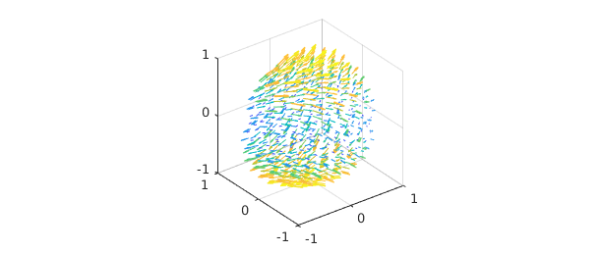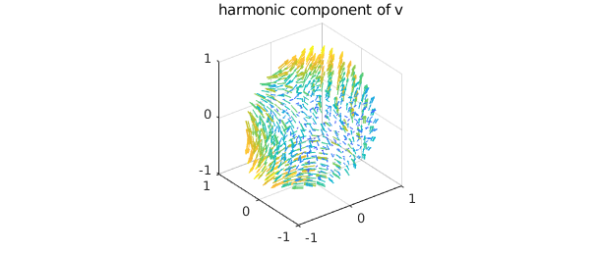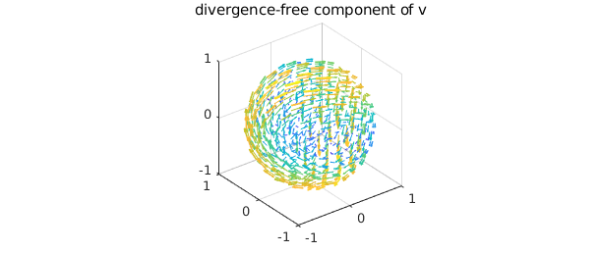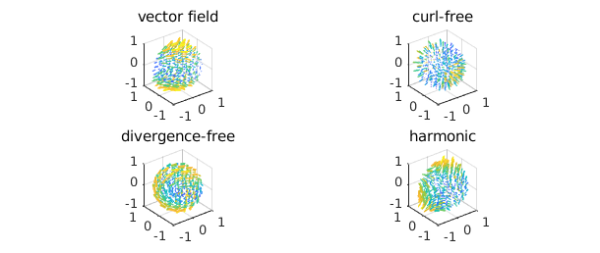### The Helmholtz-Hodge decomposition

In the subject of vector calculus, Helmholtz's theorem states that any sufficiently smooth function in the unit ball can be expressed as a sum of a curl-free, a divergence-free, and a harmonic vector field . In this Example, we show how this decomposition is computed by Ballfun and introduce the command HelmholtzDecomposition .

Let $v$ be a vector field defined in the unit ball. Helmholtz's theorem says that we can decompose $v$ as follows: $$\mathbf{v} = \nabla f + \nabla \times \psi + \nabla\phi,$$ where $f$ and $\phi$ are scalar-valued potential functions and $\psi$ is a vector field. The first term, $\nabla f$, is a gradient field and hence curl-free, while the second term, $\nabla\times\psi$, is solenoidal. The third term is a harmonic vector field (the vector Laplacian of $\nabla\phi$ is zero). From vector identities, one knows that the scalar field, $\phi$, is itself harmonic, i.e., $\Delta\phi=0$.

The Helmholtz-Hodge decomposition can be made unique by imposing additional constraints on $f$ and $\psi$ . The standard constraints are: (1) $f$ is zero on the boundary of the unit ball, (2) the normal component of $\psi$ on the boundary is zero, and (3) $\psi$ is divergence-free. The HelmholtzDecomposition command in Ballfun computes the decomposition under these additional constraints.

The Helmholtz-Hogde decomposition is an important tool in fluid dynamics as it is used for compressible flow visualization, in CFD simulations (to impose the incompressibility condition), and in topological analysis. A survey of applications is available in .

### Calculating the decomposition

To explain the procedure for computing the decomposition, we take the following vector field:

v = ballfunv(@(x,y,z)cos(x.*y).*z,@(x,y,z)sin(x.*z),@(x,y,z)y.*z);
quiver( v )### Computing the curl-free component

Since the divergence of a curl is zero and $\phi$ is harmonic, we know that the divergence of $\mathbf{v}$ is the Laplacian of $f$, i.e., $$\nabla \cdot \mathbf{v} = \nabla \cdot \nabla f = \nabla^2 f,$$ where the last equality holds because the divergence of the gradient is the Laplacian. Along with this, the zero Dirichlet conditions defines $f$.

f = poisson(div(v), @(lam,th)0, 50);
quiver( grad( f ) ), title('curl-free component of v')We confirm that this component is curl-free:

norm( curl( grad( f ) ) )
ans =
9.772028777653057e-14


### Computing the harmonic component

Now, one can define a vector field $v^{(1)}$ as $$v^{(1)} = v - \nabla f = \nabla \times \psi + \nabla \phi.$$ Since we have the identity $$\vec{n} \cdot (\nabla \times \psi)|_{\partial{B}} = \psi \times \vec{n}|_{\partial B} = 0$$ and we want to find the harmonic function $\phi$, we solve the Laplace equation $$\Delta \phi = 0.$$ The Neumann boundary conditions are given by $$\vec{n} \cdot \nabla \phi|_{\partial B} = \frac{\partial \phi}{\partial r}|_{\partial B} = \vec{n} \cdot v^{(1)}|_{\partial B}.$$ Therefore, we can solve for $\psi$ in the Helmholtz-Hodge decomposition as follows:

v1 = v - grad(f);
bc = dot(spherefunv.unormal,v1(1,:,:,'spherical'));
phi = helmholtz(ballfun(0), 0, bc, 50, 'neumann');
quiver( grad( phi ) ), title('harmonic component of v')We check the harmonicity of this component:

norm( laplacian( grad( phi ) ) )
ans =
3.527524381308947e-09


### Computing the divergence-free component

Let $v^{(2)}$ be the following vector field: $$v^{(2)} = v^{(1)} - \nabla \phi = \nabla \times \psi.$$ Since $v^{(2)}$ and $\psi$ are divergence-free, we can write their Poloidal-Toroidal decomposition  as $$v^{(2)} = \nabla\times\nabla\times(\mathbf{r}P_{v^{(2)}})+ \nabla\times(\mathbf{r}T_{v^{(2)}}),$$ $$\psi = \nabla\times\nabla\times(\mathbf{r}P_{\psi})+ \nabla\times(\mathbf{r}T_{\psi}),$$ where $\mathbf{r} = r\hat{r}$ and $\hat{r}$ denotes the unit radial vector in spherical coordinates. Moreover, the uniqueness of the PT decomposition and further vector identities lead us to the following system of equations for $\psi$: $$\Delta P_{\psi} = -T_{v^{(2)}}, \quad T_{\psi} = P_{v^{(2)}},$$ where $P_{\psi}$ is subjected to zero Dirichlet conditions because $\vec{n}\cdot\nabla\times(\mathbf{r}T_{\psi})|_{\partial B}=0$. Therefore, we can solve for $\psi$ in the Helmholtz-Hodge decomposition as follows:

v2 = v1 - grad(phi);
[Pv, Tv] = PTdecomposition(v2);
Ppsi = poisson(-Tv, @(lam,th)0, 50);
Tpsi = Pv;

We then recover the divergence-free vector field $\psi$ since $$\psi = \nabla\times\nabla\times(\mathbf{r}P_{\psi})+ \nabla\times(\mathbf{r}T_{\psi}).$$

psi = ballfunv.PT2ballfunv(Ppsi,Tpsi);
quiver( curl( psi ) ), title('divergence-free component of v')By vector identities this component is divergence-free:

norm( div( curl( psi ) ) )
ans =
1.536895357752616e-10


### Visualizing the decomposition

Here are plots of each component of the decomposition:

subplot(2,2,1)
quiver( v ,'numpts',20), title('vector field')
subplot(2,2,2)
quiver( grad(f) ,'numpts',20), title('curl-free')
subplot(2,2,3)
quiver( curl(psi) ,'numpts',20), title('divergence-free')
subplot(2,2,4)
quiver( grad(phi) ,'numpts',20), title('harmonic')As a sanity check we confirm that the decomposition has been successful:

w = grad( f ) + curl( psi ) + grad( phi );
norm( v - w )
ans =
7.815499404544524e-12


### The HelmholtzDecomposition command

Ballfun has a command HelmholtzDecomposition that computes the Helmholtz-Hodge decomposition of a vector field. Therefore, this example can be replicated with the following code:

[f, Ppsi, Tpsi, phi] = HelmholtzDecomposition( v );
psi = ballfunv.PT2ballfunv(Ppsi, Tpsi);

 G. Backus, Poloidal and toroidal fields in geomagnetic field modelling, Reviews of Geophysics, 24 (1986), pp. 75-109.

 H. Bhatia, G. Norgard, V. Pascucci, and P.-T. Bremer, The Helmholtz-Hodge decomposition--A survey, IEEE Trans. Vis. Comput. Graphics, 19 (2013), pp. 1386-1404.

 N. Boullé and A. Townsend, Computing with functions on the ball, in preparation.

 Y. Tong, S. Lombeyda, A. Hirani, and M. Desbrun, Discrete multiscale vector field decomposition, ACM Trans. Graphics, 22 (2003), pp. 445-452.